# Thevenin's Theorem

Any combination of batteries and resistances with two terminals can be replaced by a single voltage source e and a single series resistor r. The value of e is the open circuit voltage at the terminals, and the value of r is e divided by the current with the terminals short circuited.Thevenin voltage Thevenin resistance Numerical example Norton equivalent
 to AC Version Application in Digital to Analog Converter
Index

DC Circuits

 HyperPhysics***** Electricity and Magnetism R Nave
Go Back

# Thevenin Voltage

The Thevenin voltage e used in Thevenin's Theorem is an ideal voltage source equal to the open circuit voltage at the terminals. In the example below, the resistance R2 does not affect this voltage and the resistances R1 and R3 form a voltage divider, giving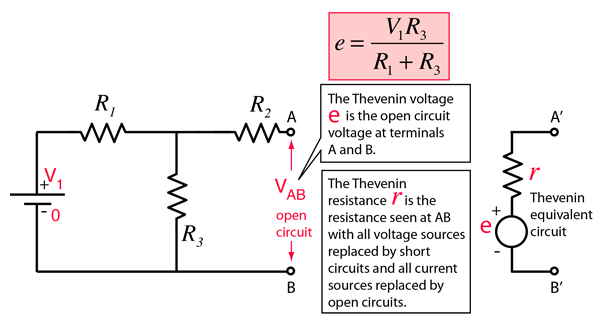Thevenin resistance Numerical example Norton equivalent
Index

DC Circuits

 HyperPhysics***** Electricity and Magnetism R Nave
Go Back

# Thevenin/Norton Resistance

The Thevenin resistance r used in Thevenin's Theorem is the resistance measured at terminals AB with all voltage sources replaced by short circuits and all current sources replaced by open circuits. It can also be calculated by dividing the open circuit voltage by the short circuit current at AB, but the previous method is usually preferable and gives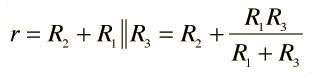The same resistance is used in the Norton equivalent.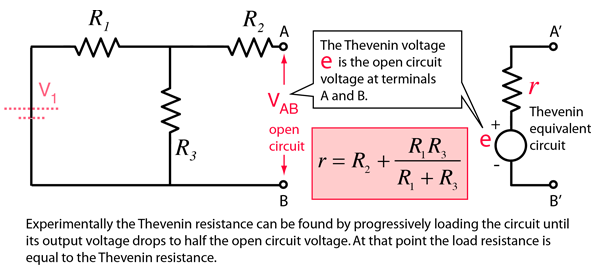Thevenin voltage Numerical example Norton equivalent
Index

DC Circuits

 HyperPhysics***** Electricity and Magnetism R Nave
Go Back

# Thevenin Example

Replacing a network by its Thevenin equivalent can simplify the analysis of a complex circuit. In this example, the Thevenin voltage is just the output of the voltage divider formed by R1 and R3. The Thevenin resistance is the resistance looking back from AB with V1 replaced by a short circuit.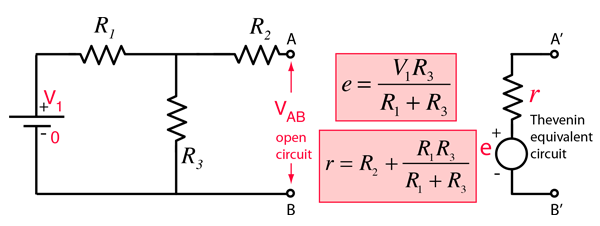For R1 =Ω, R2 = Ω, R3 =Ω,

 and voltage V1 = V

 the Thevenin voltage is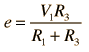V
 since R1 and R3 form a simple voltage divider.

 The Thevenin resistance is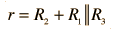= Ω.
 Apply to two-loop problem AC example Norton equivalent
Index

DC Circuits

 HyperPhysics***** Electricity and Magnetism R Nave
Go Back